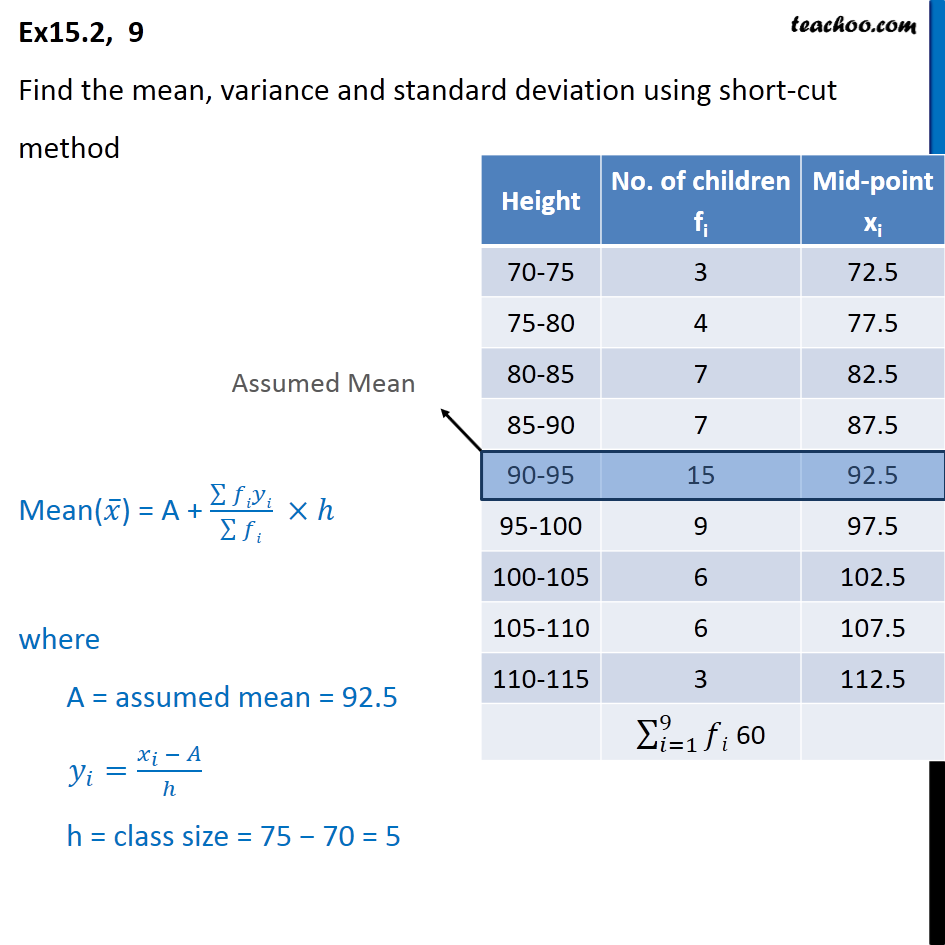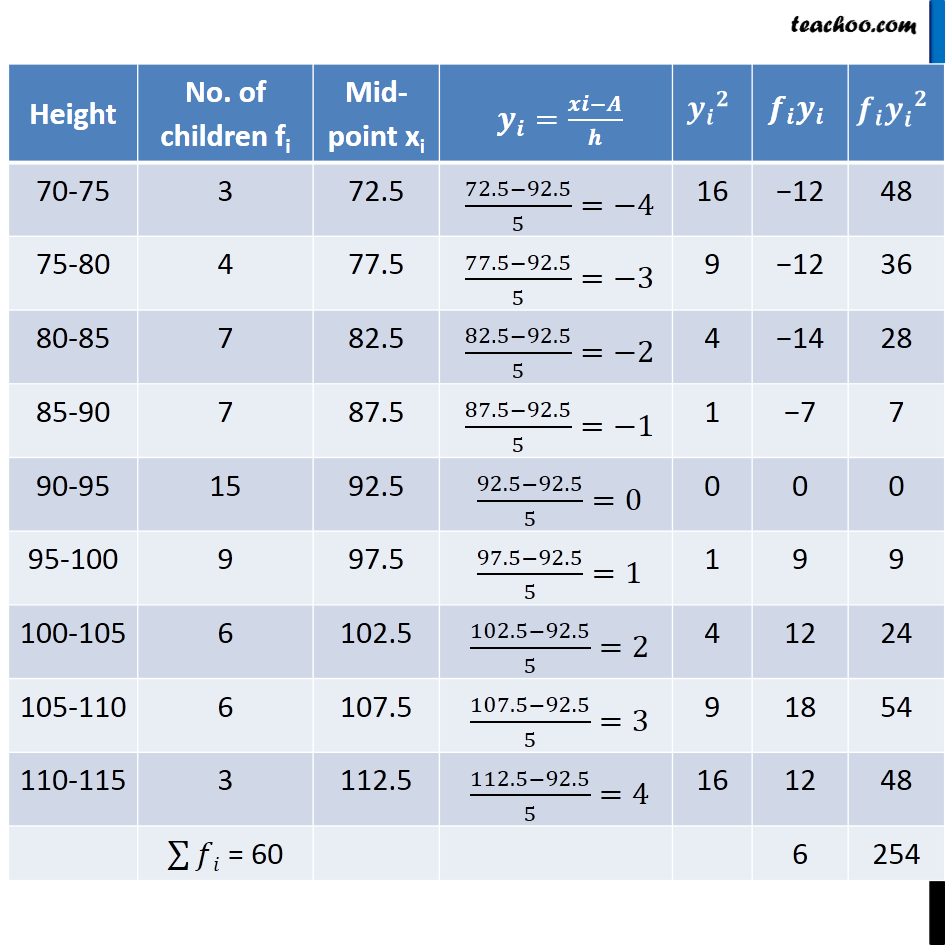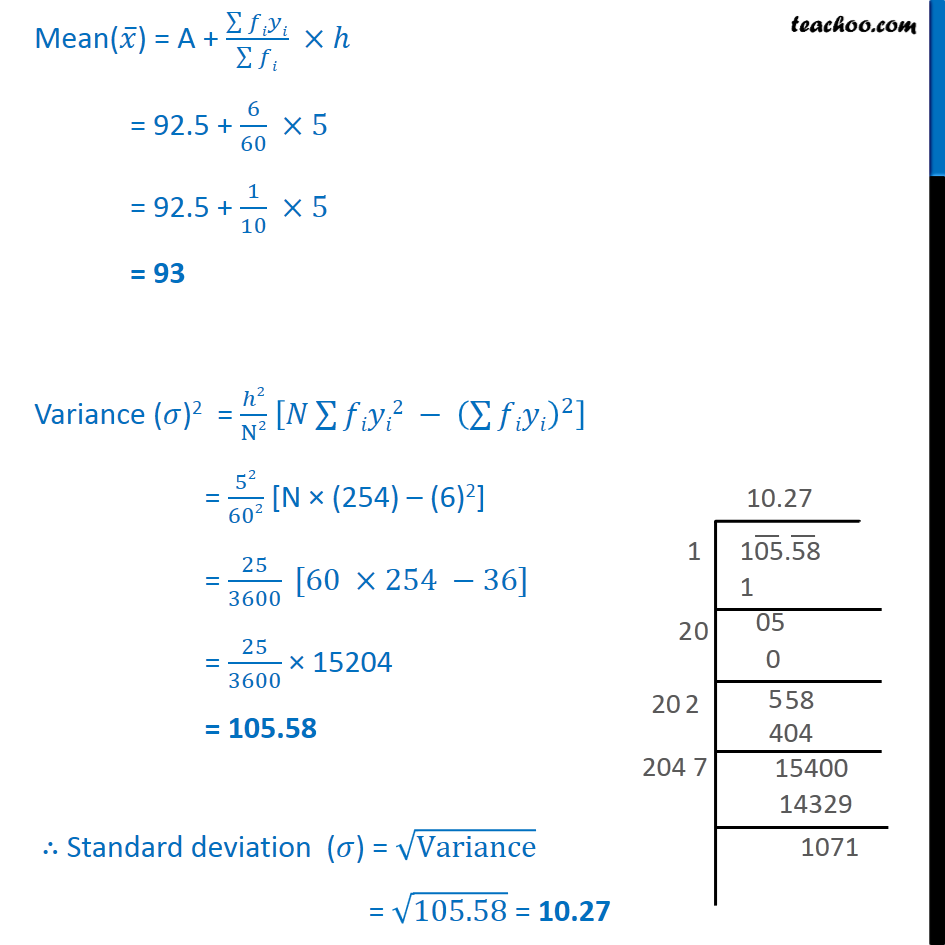Ex 13.2

Chapter 13 Class 11 Statistics
Serial order wiseLearn in your speed, with individual attention - Teachoo Maths 1-on-1 Class

### Transcript

Ex15.2, 9 Find the mean, variance and standard deviation using short-cut method Mean(𝑥 ̅) = A + (∑▒𝑓𝑖𝑦𝑖)/(∑▒𝑓𝑖) ×ℎ where A = assumed mean = 92.5 𝑦_𝑖=(𝑥_𝑖 − 𝐴)/ℎ h = class size = 75 − 70 = 5 Variance (𝜎)2 = ℎ2/N2 [𝑁∑▒𝑓𝑖𝑦𝑖2 − (∑▒𝑓𝑖𝑦𝑖)^2 ] = 52/602 [N × (254) – (6)2] = 25/3600 [60 ×254 −36] = 25/3600 × 15204 = 105.58 ∴ Standard deviation (𝜎) = √Variance = √105.58 = 10.27